# Python图表绘制：matplotlib绘图库入门

+关注继续查看

matplotlib 是python最著名的绘图库，它提供了一整套和matlab相似的命令API，十分适合交互式地行制图。而且也可以方便地将它作为绘图控件，嵌入GUI应用程序中。

2. 面向对象画图

4. 在图表中显示中文

6. 对数坐标轴

7. 学习资源

Matplotlib.pyplot快速绘图

matplotlib实际上是一套面向对象的绘图库，它所绘制的图表中的每个绘图元素，例如线条Line2D、文字Text、刻度等在内存中都有一个对象与之对应。

plt.plot()实际上会通过plt.gca()获得当前的Axes对象ax，然后再调用ax.plot()方法实现真正的绘图。

matplotlib所绘制的图表的每个组成部分都和一个对象对应，我们可以通过调用这些对象的属性设置方法set_*()或者pyplot模块的属性设置函数setp()设置它们的属性值。

matplotlib将这些缺省配置保存在一个名为“matplotlibrc”的配置文件中，通过修改配置文件，我们可以修改图表的缺省样式。配置文件的读入可以使用rc_params()，它返回一个配置字典；在matplotlib模块载入时会调用rc_params()，并把得到的配置字典保存到rcParams变量中；matplotlib将使用rcParams字典中的配置进行绘图；用户可以直接修改此字典中的配置，所做的改变会反映到此后创建的绘图元素。

Matplotlib 里的常用类的包含关系为 Figure -> Axes -> (Line2D, Text, etc.)一个Figure对象可以包含多个子图(Axes)，在matplotlib中用Axes对象表示一个绘图区域，可以理解为子图。

subplot(numRows, numCols, plotNum)

subplot将整个绘图区域等分为numRows行* numCols列个子区域，然后按照从左到右，从上到下的顺序对每个子区域进行编号，左上的子区域的编号为1。如果numRows，numCols和plotNum这三个数都小于10的话，可以把它们缩写为一个整数，例如subplot(323)和subplot(3,2,3)是相同的。subplot在plotNum指定的区域中创建一个轴对象。如果新创建的轴和之前创建的轴重叠的话，之前的轴将被删除。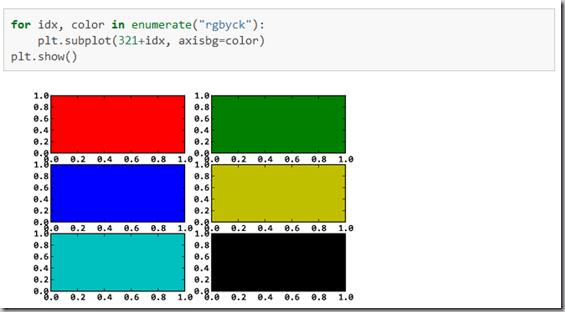subplot()返回它所创建的Axes对象，我们可以将它用变量保存起来，然后用sca()交替让它们成为当前Axes对象，并调用plot()在其中绘图。

import numpy as np
import matplotlib.pyplot as plt

plt.figure(1) # 创建图表1
plt.figure(2) # 创建图表2
ax1 = plt.subplot(211) # 在图表2中创建子图1
ax2 = plt.subplot(212) # 在图表2中创建子图2

x = np.linspace(0, 3, 100)
for i in xrange(5):
    plt.figure(1)  # # 选择图表1
    plt.plot(x, np.exp(i*x/3))
    plt.sca(ax1)   # # 选择图表2的子图1
    plt.plot(x, np.sin(i*x))
    plt.sca(ax2)  # 选择图表2的子图2
    plt.plot(x, np.cos(i*x))

plt.show()matplotlib的缺省配置文件中所使用的字体无法正确显示中文。为了让图表能正确显示中文，可以有几种解决方案。

1. 在程序中直接指定字体。

2. 在程序开头修改配置字典rcParams。

3. 修改配置文件。

matplotlib输出图象的中文显示问题

matplotlib API包含有三层，Artist层处理所有的高层结构，例如处理图表、文字和曲线等的绘制和布局。通常我们只和Artist打交道，而不需要关心底层的绘制细节。

• 创建Figure对象

• 用Figure对象创建一个或者多个Axes或者Subplot对象

• 调用Axies等对象的方法创建各种简单类型的Artists

import matplotlib.pyplot as plt



X1 = range(0, 50) Y1 = [num**2 for num in X1] # y = x^2 X2 = [0, 1] Y2 = [0, 1] # y = x



Fig = plt.figure(figsize=(8,4)) # Create a figure' instance Ax = Fig.add_subplot(111) # Create a axes' instance in the figure Ax.plot(X1, Y1, X2, Y2) # Create a Line2D instance in the axes



Fig.show() Fig.savefig("test.pdf")

《Python科学计算》(Numpy视频matplotlib-绘制精美的图表(快速绘图)(面向对象绘图)（深入浅出适合系统学习）

Matplotlib.pylab快速绘图

matplotlib还提供了一个名为pylab的模块，其中包括了许多NumPy和pyplot模块中常用的函数，方便用户快速进行计算和绘图，十分适合在IPython交互式环境中使用。这里使用下面的方式载入pylab模块：

>>> import pylab as pl

1 安装numpy和matplotlib

>>> import numpy
>>> numpy.__version__

>>> import matplotlib
>>> matplotlib.__version__

2 两种常用图类型：Line and scatter plots(使用plot()命令), histogram(使用hist()命令)

2.1 折线图&散点图 Line and scatter plots

2.1.1 折线图 Line plots(关联一组x和y值的直线)

import numpy as np
import pylab as pl

x = [1, 2, 3, 4, 5]# Make an array of x values
y = [1, 4, 9, 16, 25]# Make an array of y values for each x value

pl.plot(x, y)# use pylab to plot x and y
pl.show()# show the plot on the screen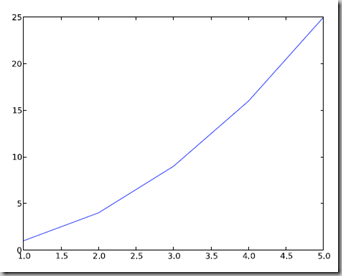2.1.2 散点图 Scatter plots

2.2  美化 Making things look pretty

2.2.1 线条颜色 Changing the line color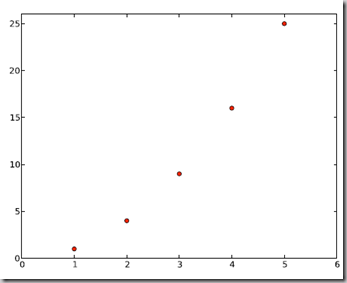2.2.2 线条样式 Changing the line style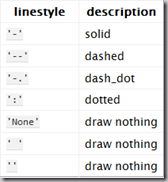2.2.3 marker样式 Changing the marker style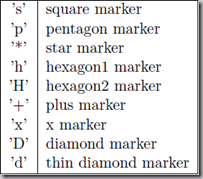2.2.4 图和轴标题以及轴坐标限度 Plot and axis titles and limits

import numpy as np
import pylab as pl

x = [1, 2, 3, 4, 5]# Make an array of x values
y = [1, 4, 9, 16, 25]# Make an array of y values for each x value
pl.plot(x, y)# use pylab to plot x and y

pl.title(’Plot of y vs. x’)# give plot a title
pl.xlabel(’x axis’)# make axis labels
pl.ylabel(’y axis’)

pl.xlim(0.0, 7.0)# set axis limits
pl.ylim(0.0, 30.)

pl.show()# show the plot on the screen2.2.5 在一个坐标系上绘制多个图 Plotting more than one plot on the same set of axes

import numpy as np
import pylab as pl

x1 = [1, 2, 3, 4, 5]# Make x, y arrays for each graph
y1 = [1, 4, 9, 16, 25]
x2 = [1, 2, 4, 6, 8]
y2 = [2, 4, 8, 12, 16]

pl.plot(x1, y1, ’r’)# use pylab to plot x and y
pl.plot(x2, y2, ’g’)

pl.title(’Plot of y vs. x’)# give plot a title
pl.xlabel(’x axis’)# make axis labels
pl.ylabel(’y axis’)


pl.xlim(0.0, 9.0)# set axis limits
pl.ylim(0.0, 30.)


pl.show()# show the plot on the screen2.2.6  图例 Figure legends

pl.legend((plot1, plot2), (’label1, label2’), 'best’, numpoints=1)

import numpy as np
import pylab as pl

x1 = [1, 2, 3, 4, 5]# Make x, y arrays for each graph
y1 = [1, 4, 9, 16, 25]
x2 = [1, 2, 4, 6, 8]
y2 = [2, 4, 8, 12, 16]

plot1 = pl.plot(x1, y1, ’r’)# use pylab to plot x and y : Give your plots names
plot2 = pl.plot(x2, y2, ’go’)

pl.title(’Plot of y vs. x’)# give plot a title
pl.xlabel(’x axis’)# make axis labels
pl.ylabel(’y axis’)


pl.xlim(0.0, 9.0)# set axis limits
pl.ylim(0.0, 30.)


pl.legend([plot1, plot2], (’red line’, ’green circles’), ’best’, numpoints=1)# make legend
pl.show()# show the plot on the screen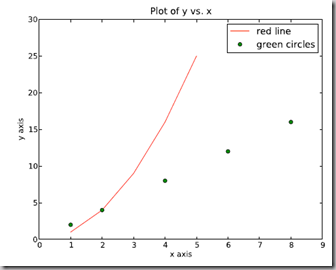2.3 直方图 Histograms

import numpy as np
import pylab as pl

# make an array of random numbers with a gaussian distribution with
# mean = 5.0
# rms = 3.0
# number of points = 1000
data = np.random.normal(5.0, 3.0, 1000)

# make a histogram of the data array
pl.hist(data)

# make plot labels
pl.xlabel(’data’)
pl.show()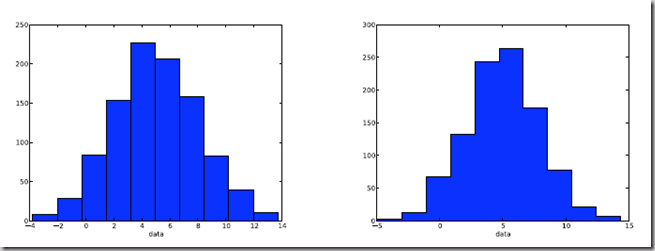2.3.1 自定义直方图bin宽度 Setting the width of the histogram bins manually

bins = np.arange(-5., 16., 1.) #浮点数版本的range
pl.hist(data, bins, histtype=’stepfilled’)3 同一画板上绘制多幅子图 Plotting more than one axis per canvas

fig1 = pl.figure(1)
pl.subplot(211)
subplot(211)把绘图区域等分为2行*1列共两个区域, 然后在区域1(上区域)中创建一个轴对象. pl.subplot(212)在区域2(下区域)创建一个轴对象。You can play around with plotting a variety of layouts. For example, Fig. 11 is created using the following commands:

f1 = pl.figure(1)
pl.subplot(221)
pl.subplot(222)
pl.subplot(212)4 绘制文件中的数据Plotting data contained in files

4.1 从Ascii文件中读取数据 Reading data from ascii files

**********************************************

# fakedata.txt
0 0
1 1
2 4
3 9
4 16
5 25
6 36
7 49
8 64
9 81
0 0
1 1
2 4
3 9
4 16
5 25
6 36
7 49
8 64
9 81

**********************************************

import numpy as np
import pylab as pl

# Use numpy to load the data contained in the file
# ’fakedata.txt’ into a 2-D array called data
data = np.loadtxt(’fakedata.txt’)

# plot the first column as x, and second column as y
pl.plot(data[:,0], data[:,1], ’ro’)
pl.xlabel(’x’)
pl.ylabel(’y’)
pl.xlim(0.0, 10.)
pl.show()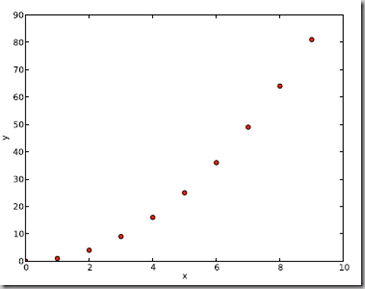4.2 写入数据到文件 Writing data to a text file

import numpy as np
# Let’s make 2 arrays (x, y) which we will write to a file
# x is an array containing numbers 0 to 10, with intervals of 1
x = np.arange(0.0, 10., 1.)
# y is an array containing the values in x, squared
y = x*x
print ’x = ’, x
print ’y = ’, y

# Now open a file to write the data to
# ’w’ means open for ’writing’
file = open(’testdata.txt’, ’w’)
# loop over each line you want to write to file
for i in range(len(x)):
    # make a string for each line you want to write
    # ’\t’ means ’tab’
    # ’\n’ means ’newline’
    # ’str()’ means you are converting the quantity in brackets to a string type
    txt = str(x[i]) + ’\t’ + str(y[i]) + ’ \n’
    # write the txt to the file
    file.write(txt)
# Close your file
file.close()

Matlplotlib对LaTeX有一定的支持，如果记得使用raw字符串语法会很自然：

xlabel(r"[Math Processing Error]")

Here is a simple example:

# plain text
plt.title('alpha > beta')

produces “alpha > beta”.

Whereas this:produces "".

import matplotlib.pyplot as plt

x = arange(1,1000,1)
r = -2
c = 5
y = [5*(a**r) for a in x]

fig = plt.figure()

ax.loglog(x,y,label = r"[Math Processing Error]")
ax.legend()
ax.set_xlabel(r"x")
ax.set_ylabel(r"y")

plt.plot(x,y,label="[Math Processing Error]",color="red",linewidth=2)
plt.plot(x,z,"b--",label="[Math Processing Error]")

plot函数的调用方式很灵活，第一句将x,y数组传递给plot之后，用关键字参数指定各种属性：

• label : 给所绘制的曲线一个名字，此名字在图示(legend)中显示。只要在字符串前后添加"$"符号，matplotlib就会使用其内嵌的latex引擎绘制的数学公式。 • color : 指定曲线的颜色 • linewidth : 指定曲线的宽度 详细的可以参考matplotlib官方教程: Writing mathematical expressions Text rendering With LaTeX 有几个问题： • matplotlib.rcParams属性字典 • 想要它正常工作，在matplotlibrc配置文件中需要设置text.markup = "tex"。 • 如果你希望图表中所有的文字（包括坐标轴刻度标记）都是LaTeX'd,需要在matplotlibrc中设置text.usetex = True。如果你使用LaTeX撰写论文，那么这一点对于使图表和论文中其余部分保持一致是很有用的。 matplotlib使用小结 LaTeX科技排版 参考文献自动搜集管理完美攻略（图文版）:Latex+Lyx+Zotero 对数坐标轴 在实际中，我们可能经常会用到对数坐标轴，这时可以用下面的三个函数来实现 ax.semilogx(x,y) #x轴为对数坐标轴 ax.semilogy(x,y) #y轴为对数坐标轴 ax.loglog(x,y) #双对数坐标轴 学习资源 Gnuplot的介绍 官方英文资料： IBM:基于 Python Matplotlib 模块的高质量图形输出(2005年的文章有点旧) matplotlib技巧集(绘制不连续函数的不连续点；参数曲线上绘制方向箭头；修改缺省刻度数目；Y轴不同区间使用不同颜色填充的曲线区域。) Python:使用matp绘制不连续函数的不连续点；参数曲线上绘制方向箭头；修改缺省刻度数目；Y轴不同区间使用不同颜色填充的曲线区域。lotlib绘制图表 matplotlib图表中图例大小及字体相关问题 本文转自 chengxuyonghu 51CTO博客，原文链接：http://blog.51cto.com/6226001001/1613005，如需转载请自行联系原作者 版权声明：本文内容由阿里云实名注册用户自发贡献，版权归原作者所有，阿里云开发者社区不拥有其著作权，亦不承担相应法律责任。具体规则请查看《阿里云开发者社区用户服务协议》和《阿里云开发者社区知识产权保护指引》。如果您发现本社区中有涉嫌抄袭的内容，填写侵权投诉表单进行举报，一经查实，本社区将立刻删除涉嫌侵权内容。 作者高分内容 更多 相关文章如何设置阿里云服务器安全组？阿里云安全组规则详细解说 阿里云安全组设置详细图文教程（收藏起来） 阿里云服务器安全组设置规则分享，阿里云服务器安全组如何放行端口设置教程。阿里云会要求客户设置安全组，如果不设置，阿里云会指定默认的安全组。那么，这个安全组是什么呢？顾名思义，就是为了服务器安全设置的。安全组其实就是一个虚拟的防火墙，可以让用户从端口、IP的维度来筛选对应服务器的访问者，从而形成一个云上的安全域。 19225 0阿里云服务器如何登录？阿里云服务器的三种登录方法 购买阿里云ECS云服务器后如何登录？场景不同，阿里云优惠总结大概有三种登录方式： 登录到ECS云服务器控制台 在ECS云服务器控制台用户可以更改密码、更换系. 28485 0阿里云服务器设置教程：安装宝塔面板一步到位管理你的ECS 63658 0阿里云服务器安全组设置内网互通的方法 虽然0.0.0.0/0使用非常方便，但是发现很多同学使用它来做内网互通，这是有安全风险的，实例有可能会在经典网络被内网IP访问到。下面介绍一下四种安全的内网互联设置方法。 购买前请先：领取阿里云幸运券，有很多优惠，可到下文中领取。 22259 0Xshell使用SSH远程登录阿里云ECS服务器CentOS7 22030 0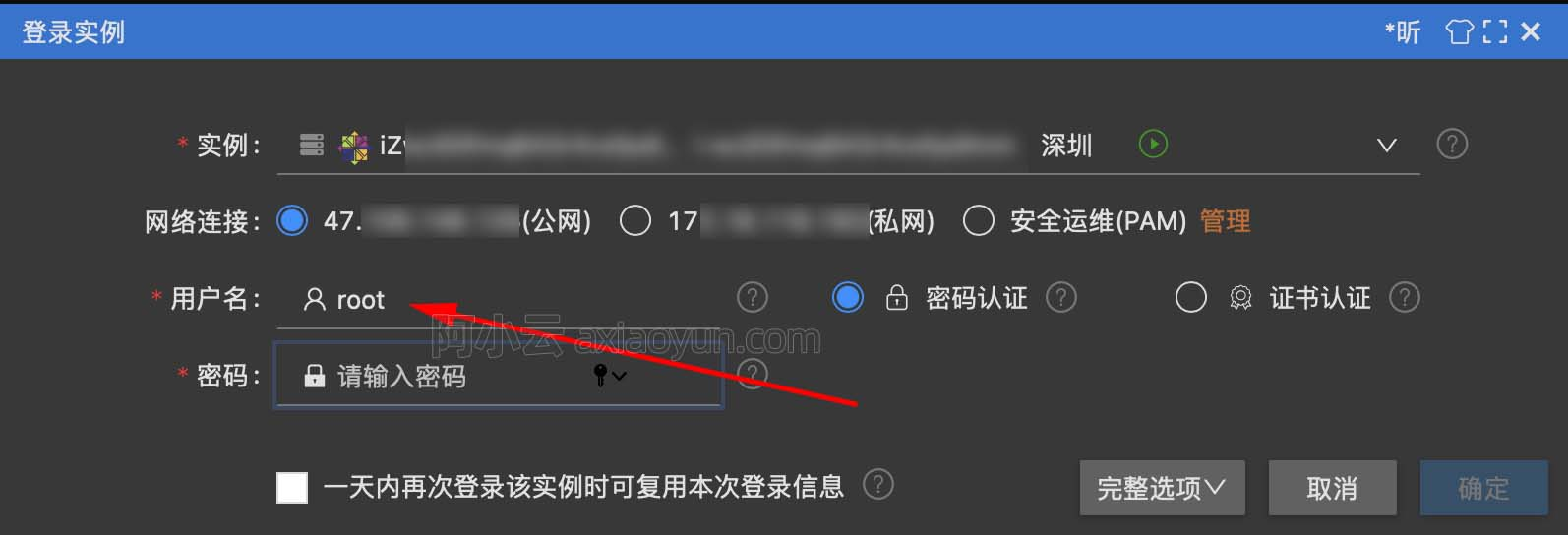阿里云服务器ECS登录用户名是什么？系统不同默认账号也不同 阿里云服务器Windows系统默认用户名administrator，Linux镜像服务器用户名root 15910 0阿里云服务器端口号设置 阿里云服务器初级使用者可能面临的问题之一. 使用tomcat或者其他服务器软件设置端口号后,比如 一些不是默认的, mysql的 3306, mssql的1433,有时候打不开网页, 原因是没有在ecs安全组去设置这个端口号. 解决: 点击ecs下网络和安全下的安全组 在弹出的安全组中,如果没有就新建安全组,然后点击配置规则 最后如上图点击添加...或快速创建. have fun! 将编程看作是一门艺术，而不单单是个技术。 20365 0云服务器 ECS 配置：ECS之Windows服务器时钟同步设置 15904 0腾讯云服务器 设置ngxin + fastdfs +tomcat 开机自启动 在tomcat中新建一个可以启动的 .sh 脚本文件 /usr/local/tomcat7/bin/ export JAVA_HOME=/usr/local/java/jdk7 export PATH=$JAVA_HOME/bin/:\$PATH export CLASSPATH=.
14884 023546 0
20378

0

JS零基础入门教程（上册）